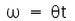NEET  >  Test: Kinematic Variables in Rotation

# Test: Kinematic Variables in Rotation

Test Description

## 10 Questions MCQ Test Physics Class 11 | Test: Kinematic Variables in Rotation

Test: Kinematic Variables in Rotation for NEET 2023 is part of Physics Class 11 preparation. The Test: Kinematic Variables in Rotation questions and answers have been prepared according to the NEET exam syllabus.The Test: Kinematic Variables in Rotation MCQs are made for NEET 2023 Exam. Find important definitions, questions, notes, meanings, examples, exercises, MCQs and online tests for Test: Kinematic Variables in Rotation below.
Solutions of Test: Kinematic Variables in Rotation questions in English are available as part of our Physics Class 11 for NEET & Test: Kinematic Variables in Rotation solutions in Hindi for Physics Class 11 course. Download more important topics, notes, lectures and mock test series for NEET Exam by signing up for free. Attempt Test: Kinematic Variables in Rotation | 10 questions in 10 minutes | Mock test for NEET preparation | Free important questions MCQ to study Physics Class 11 for NEET Exam | Download free PDF with solutions
 1 Crore+ students have signed up on EduRev. Have you?
Test: Kinematic Variables in Rotation - Question 1

### Which of the following is the unit of angular acceleration?

Detailed Solution for Test: Kinematic Variables in Rotation - Question 1

Angular acceleration is the rate of change of angular velocity. In three dimensions, it is a pseudo-vector. In SI units, it is measured in radians per second squared (rad/s2), and is usually denoted by the Greek letter alpha (α).

Test: Kinematic Variables in Rotation - Question 2

### Which of the following statements give the relationship between linear velocity and angular velocity correctly?

Detailed Solution for Test: Kinematic Variables in Rotation - Question 2

Linear velocity (V) = angular velocity (w) × radius (r)
v = w × r
w = v/r

Test: Kinematic Variables in Rotation - Question 3

### The angular velocity of the second hand of a clock is :

Detailed Solution for Test: Kinematic Variables in Rotation - Question 3

Second’s hand of a clock completes one turn in one second.i.e 1 revolution per minute

Angular velocity of second’s hand;

ω = 2π/60 = π/30 rad/s

Test: Kinematic Variables in Rotation - Question 4

Vectorially, angular velocity of a rotating body is represented :

Detailed Solution for Test: Kinematic Variables in Rotation - Question 4

Angular velocity is a pseudovector , with its magnitude measuring the rate of rotation and its direction pointing along the axis of rotation ( perpendicular to radius and velocity vectors ) .

Test: Kinematic Variables in Rotation - Question 5

A man standing on a table rotating about the central axis pulls his hands holding the dumb bells towards chest. How the angular velocity will change?

Detailed Solution for Test: Kinematic Variables in Rotation - Question 5

When the man pulls his hands towards chest, the moment of inertia will decrease and due to conservation of angular momentum, angular velocity will increase.

Test: Kinematic Variables in Rotation - Question 6

Angle of line relative to a fixed direction is

Detailed Solution for Test: Kinematic Variables in Rotation - Question 6

Angle of line relative to a fixed direction is angular position.

Test: Kinematic Variables in Rotation - Question 7

A body is moving with a constant speed v in a circle of radius r. What is the angular acceleration?

Detailed Solution for Test: Kinematic Variables in Rotation - Question 7

When a body performs circular motion it has got two accelerations radial acc. And tangential acc. Radial acc. Is responsible for changing the direction of velocity of body but tangential acc. Is responsible for changing the magnitide of velocity of body. As the body is moving with constant speed so tangential acc. Is zero. As we know that tangential acc. = radius * angular acc. So angular acc.is zero.

Test: Kinematic Variables in Rotation - Question 8

What is the number of instantaneous centres of rotation for a 6-link mechanism?

Detailed Solution for Test: Kinematic Variables in Rotation - Question 8

N = n(n – 1)/2 = 6 x (6 – 1)/2 = 15.

Test: Kinematic Variables in Rotation - Question 9

Four billiards balls, each of mass 0.5 kg, all are travelling in the same direction on a billiard table, with speeds of 2 m/s, 4 m/s, 8 m/s and 10 m/s. What is the linear momentum of this system?

Detailed Solution for Test: Kinematic Variables in Rotation - Question 9

As the direction of all the four velocities are the same thus their momentum also have same directions and hence they can be directly added.
Thus total momentum of the system, P = 0.5 x 2 + 0.5 x 4 + 0.5 x 8 + 0.5 x 10
= 1 + 2 + 4 + 5
= 12 kg m /sec

Test: Kinematic Variables in Rotation - Question 10

Which of the following relations is wrong?

Detailed Solution for Test: Kinematic Variables in Rotation - Question 10

Just like the equations of motion in 1D, we get the equations of motion for rotation.
We know that s = d/t, analogous to it isHence D is the correct answer.

## Physics Class 11

124 videos|464 docs|210 tests
Information about Test: Kinematic Variables in Rotation Page
In this test you can find the Exam questions for Test: Kinematic Variables in Rotation solved & explained in the simplest way possible. Besides giving Questions and answers for Test: Kinematic Variables in Rotation, EduRev gives you an ample number of Online tests for practice

## Physics Class 11

124 videos|464 docs|210 tests

### How to Prepare for NEET

Read our guide to prepare for NEET which is created by Toppers & the best Teachers(Scan QR code)